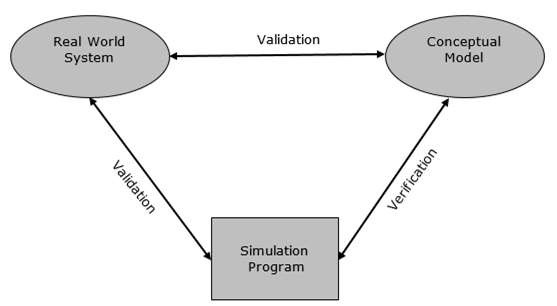# Verification & Validation

#### Creo Parametric-3D Modelling Software (Professional)

130 Lectures 16.5 hours

#### Learn Product Design and Modelling with Photoshop 3D

11 Lectures 53 mins

#### Hydraulic System Modelling with Matlab and System Dynamics

10 Lectures 2.5 hours

One of the real problems that the simulation analyst faces is to validate the model. The simulation model is valid only if the model is an accurate representation of the actual system, else it is invalid.

Validation and verification are the two steps in any simulation project to validate a model.

• Validation is the process of comparing two results. In this process, we need to compare the representation of a conceptual model to the real system. If the comparison is true, then it is valid, else invalid.

• Verification is the process of comparing two or more results to ensure its accuracy. In this process, we have to compare the model’s implementation and its associated data with the developer's conceptual description and specifications.## Verification & Validation Techniques

There are various techniques used to perform Verification & Validation of Simulation Model. Following are some of the common techniques −

### Techniques to Perform Verification of Simulation Model

Following are the ways to perform verification of simulation model −

• By using programming skills to write and debug the program in sub-programs.

• By using “Structured Walk-through” policy in which more than one person is to read the program.

• By tracing the intermediate results and comparing them with observed outcomes.

• By checking the simulation model output using various input combinations.

• By comparing final simulation result with analytic results.

### Techniques to Perform Validation of Simulation Model

Step 1 − Design a model with high validity. This can be achieved using the following steps −

• The model must be discussed with the system experts while designing.
• The model must interact with the client throughout the process.
• The output must supervised by system experts.

Step 2 − Test the model at assumptions data. This can be achieved by applying the assumption data into the model and testing it quantitatively. Sensitive analysis can also be performed to observe the effect of change in the result when significant changes are made in the input data.

Step 3 − Determine the representative output of the Simulation model. This can be achieved using the following steps −

• Determine how close is the simulation output with the real system output.

• Comparison can be performed using the Turing Test. It presents the data in the system format, which can be explained by experts only.

• Statistical method can be used for compare the model output with the real system output.

## Model Data Comparison with Real Data

After model development, we have to perform comparison of its output data with real system data. Following are the two approaches to perform this comparison.

### Validating the Existing System

In this approach, we use real-world inputs of the model to compare its output with that of the real-world inputs of the real system. This process of validation is straightforward, however, it may present some difficulties when carried out, such as if the output is to be compared to average length, waiting time, idle time, etc. it can be compared using statistical tests and hypothesis testing. Some of the statistical tests are chi-square test, Kolmogorov-Smirnov test, Cramer-von Mises test, and the Moments test.

### Validating the First Time Model

Consider we have to describe a proposed system which doesn’t exist at the present nor has existed in the past. Therefore, there is no historical data available to compare its performance with. Hence, we have to use a hypothetical system based on assumptions. Following useful pointers will help in making it efficient.

• Subsystem Validity − A model itself may not have any existing system to compare it with, but it may consist of a known subsystem. Each of that validity can be tested separately.

• Internal Validity − A model with high degree of internal variance will be rejected as a stochastic system with high variance due to its internal processes will hide the changes in the output due to input changes.

• Sensitivity Analysis − It provides the information about the sensitive parameter in the system to which we need to pay higher attention.

• Face Validity − When the model performs on opposite logics, then it should be rejected even if it behaves like the real system.